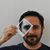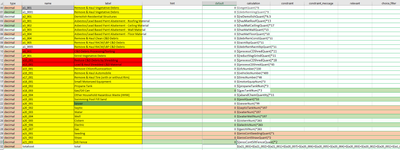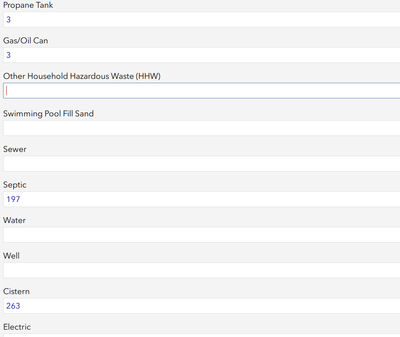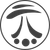# Sum Subtotals fields to get a total field error

402
3
07-29-2021 01:01 PMNew Contributor III

Hello,

I have one question.

I am doing a survey with connect.

I have some questions where i collect quantity, then those fields i multiply for cost in dollars

for example i have 3 cars then i multiply that for 500 dlls so my sub total is 1500then to calculate a total y sum all subtotals name fields as \${a21_003}+\${a21_002}+\${a21_001}+\${a20_007}+\${a20_006}+\${a20_005}+\${a20_004}+\${a20_003}+\${a20_002}+\${a20_001}+\${a1_001}+\${a1_0001}+\${a2_001}+... for the 32 records.

i have some issue. If some of my sub total field is empty my total do not work, it appear empty. so, i thought that if I put a default value it would take it as not empty but in my survey my field are empty and not with 0 as should be the default value.as you can see, my subtotal works fine but my total never works because when we collect data we do not have all of those debris to collect.

So my question is how can I solve this issue, because i need to create a report with a total cost but i am not able to generate the total field.

Thanks,

Diego Llamas

Tags (4)
1 Solution

Accepted SolutionsbyMVP Notable Contributor

Check out coalesce function for this.

coalesce(value1, value2)   Returns the first nonempty value. Supports only two values.

Use it like coalesce(\${a21_003},0)

3 RepliesbyMVP Notable Contributor

Check out coalesce function for this.

coalesce(value1, value2)   Returns the first nonempty value. Supports only two values.

Use it like coalesce(\${a21_003},0)Occasional Contributor

Hey  DougBrowning great tip. Where did you find that syntax?byMVP Notable Contributor